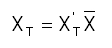﻿ Output > Tcev

# HIGH FLOOD FLOWS EVALUATION

The high flood flows' evaluation for T values (recurrence interval) can be done on hydrologic data (direct methods) and on  specific studies performed (semi empirical direct methods).

It's clear that such an important figure must be done with an uniform procedure on all national territory, so all evaluation should begin by probabilistically determination of the annual maximum flows with extreme value distribution or with a double  component (TCEV – Two Component Extreme Value).

With X is indicated the high flood's peak flow annual maximum and with XT  X' s maximum value that corresponds  to a predefined overflow period T expressed in years, so we have:

XT = KT m(X)

Where:

KT = probabilistic Seismic corrections' inertial effects growing factor, constant for homogeneous surfaces;

m(X) = X variable maximum annual distribution average;

TCEV takes into consideration the following cumulated probability function for X' s maximum annual value: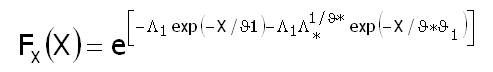where X is the considered variable (the annual peak maximum flow for t period rain) and Λ1, θ1, Λ*, θ* are the distribution parameters.

For homogeneous rainfall sub-zones the parameters Λ1, Λ*, θ* are considered constant, and the X variable is substituted with a dimensional value X’ that is obtained with:

X’ = X/ μ

Where μ is the index flow, for medium X.

With this variable the cumulative probability function can be expressed: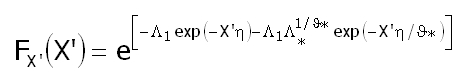with η= μ/θ1

So, for XT expression a T value must be assigned and FX’ (X’) is determined with:

FX’(X’) = 1 1/T

so, next is the estimation of X’T (fill factor).

For the practical estimations of the fill factor we can use: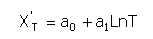- a0 and a1 are variable coefficients depending on the region.

For the estimation of the index flow μ we can appeal to the arithmetical average of the observed vales of X, or, if the data is not available, we can appeal to inter polar relations for every homogeneous region or zone with the following generic expression:

m(Q) =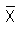= c1 AC2

with A the basin's area and c1 and c2 the parameters for each region of the various zones.

With the obtained values it's determined: